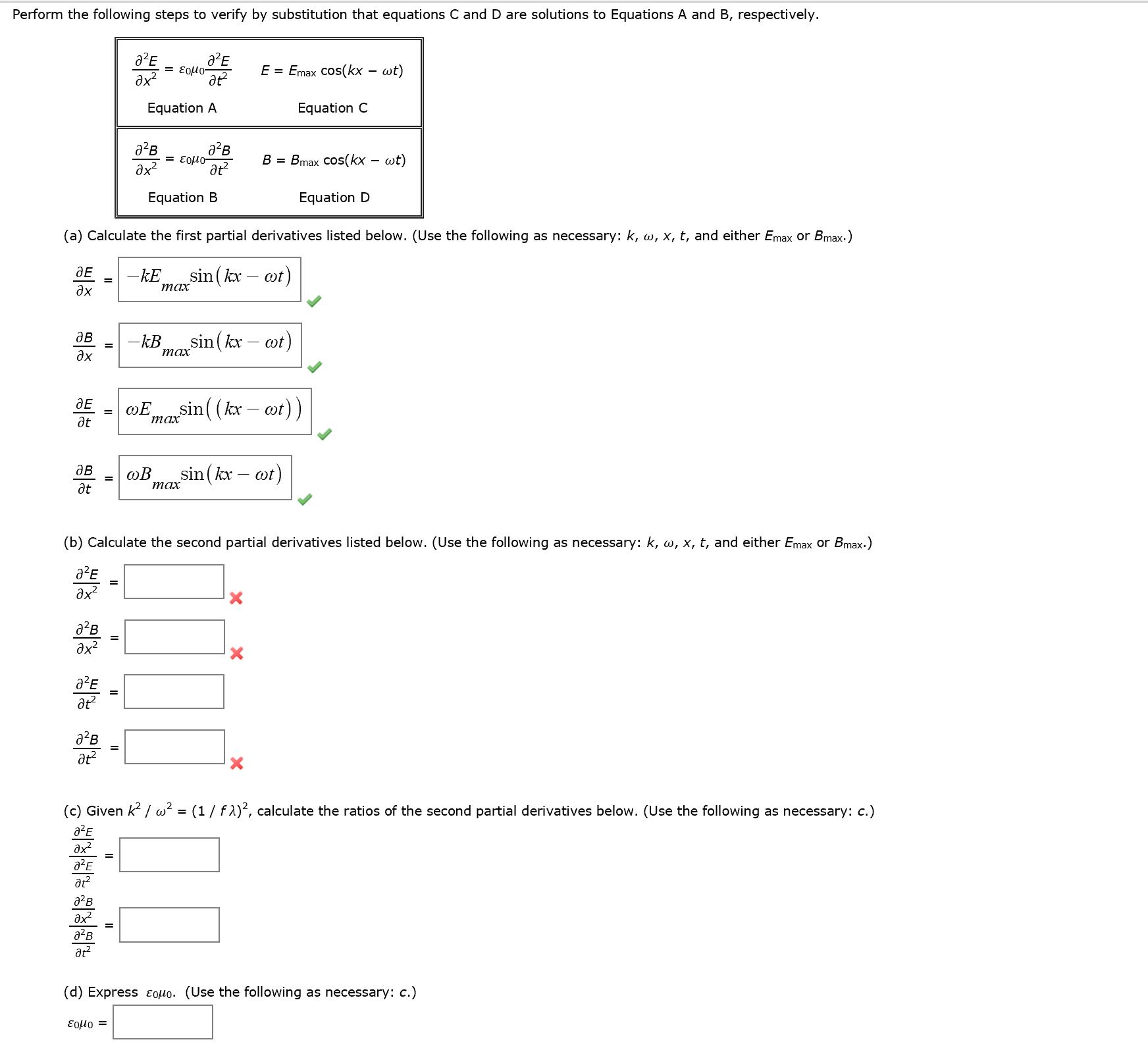# Perform the following steps to verify by substitution that C and D are solutions to equation...

###### Question:Perform the following steps to verify by substitution that C and D are solutions to equation A and B, respectively. Partial^2E/Partial x^2 = epsilon0 mu0 Partial^2E/Partial t^2 E = E_max cos(kx = omega t) Equation A Equation C Partial^2B/Partial x^2 = epsilon0 mu0 Partial^2B/Partial t^2 B = B_max cos(kx = omega t) Equation B Equation D calculate the first partial derivatives listed below. (Use the following as k, omega, x, t, and either E_max or B_max.) Partial E/Partial x = -kE_max sin(kx - omega t) Partial B/Partial x = -kB_max sin(kx - omega t) Partial E/Partial t = -omega E_max sin(kx - omega t) calculate the second partial derivatives listed below. (Use the following as k, omega, x, t, and either E_max or B_max.) Partial^2 E/Partial x^2 = Partial^2 B/Partial x^2 = Partial^2 E/Partial t^2 = Partial^2 B/Partial t^2 = Given k^2/omega^2 = (1/f lambda)^2, calculate the ratios of the second partial derivatives below. (use the following as necessary: c.) Partial^2 E/Partial x^2 = Partial^2 E/Partial t^2 = Partial^2 B/Partial x^2 = Partial^2 B/Partial t^2 = Express epsilon0 mu0. (Use the following as necessary: c.) epsilon0 mu0 =